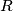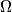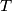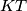### IMO Shortlist 2017 problem G2

Kvaliteta:
Avg: 0,0
Težina:
Avg: 6,0

Let$R$ and$S$ be different points on a circle$\Omega$ such that$RS$ is not a diameter. Let$\ell$ be the tangent line to$\Omega$ at$R$. Point$T$ is such that$S$ is the midpoint of the line segment$RT$. Point$J$ is chosen on the shorter arc$RS$ of$\Omega$ so that the circumcircle$\Gamma$ of triangle$JST$ intersects$\ell$ at two distinct points. Let$A$ be the common point of$\Gamma$ and$\ell$ that is closer to$R$. Line$AJ$ meets$\Omega$ again at$K$. Prove that the line$KT$ is tangent to$\Gamma$.

Izvor: https://www.imo-official.org/problems/IMO2017SL.pdf